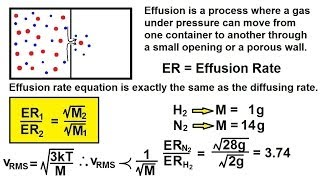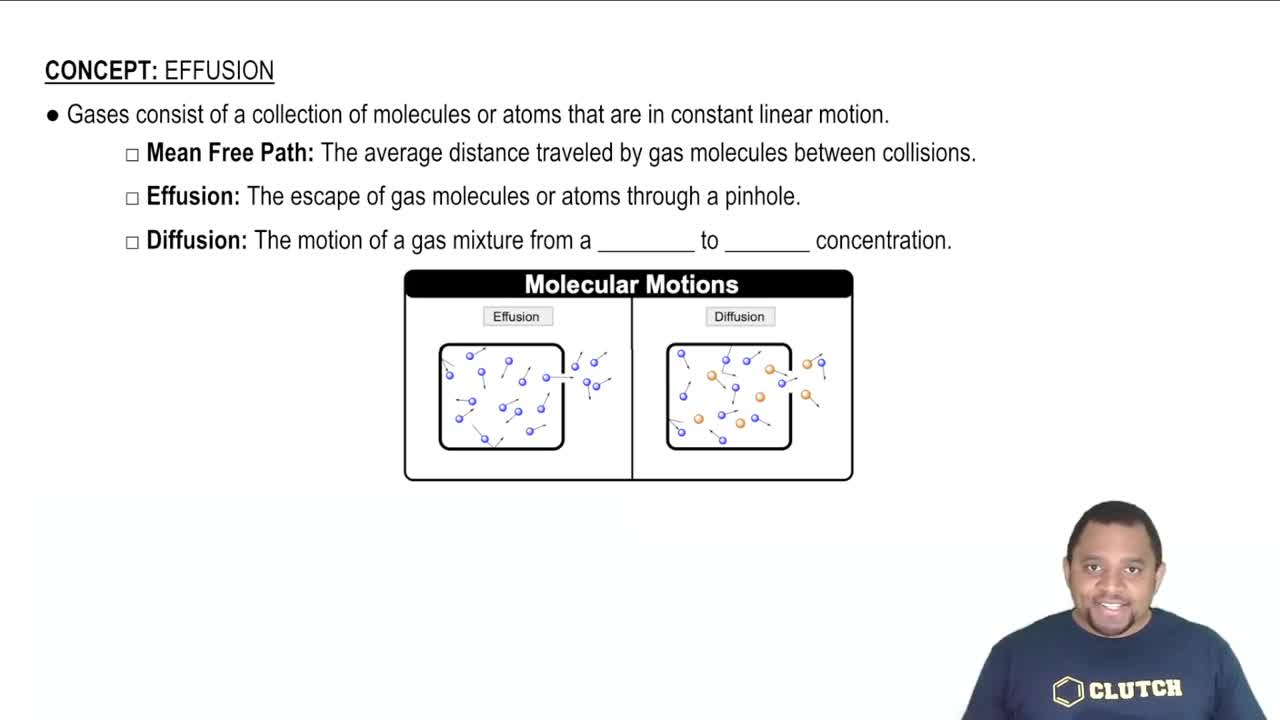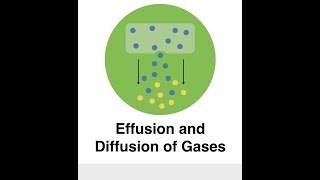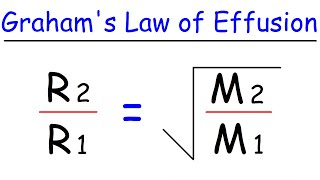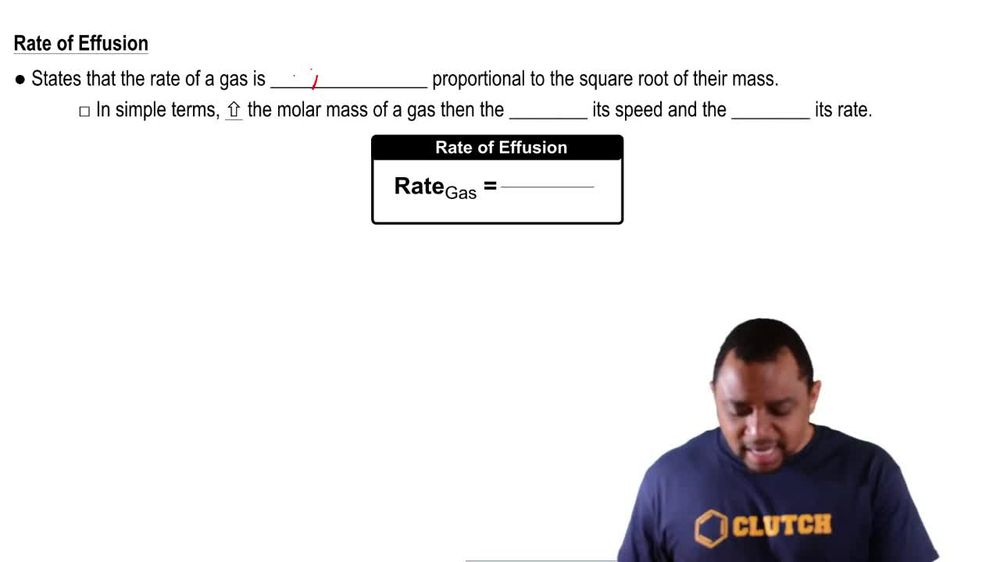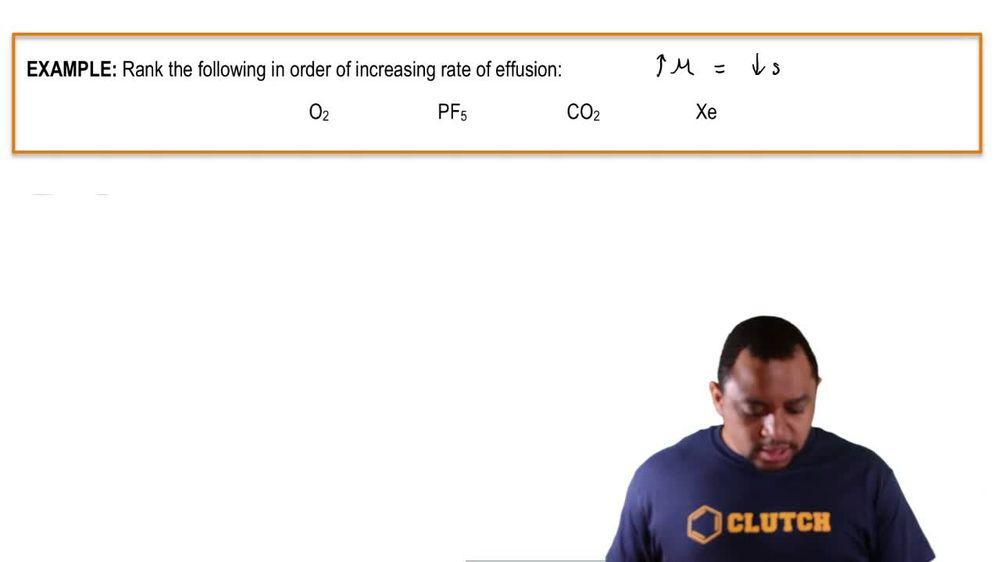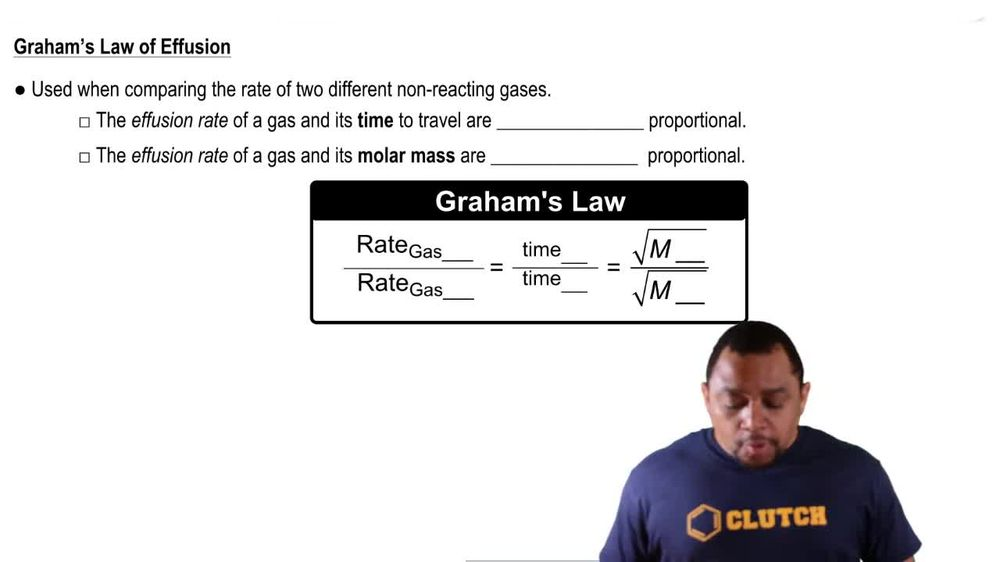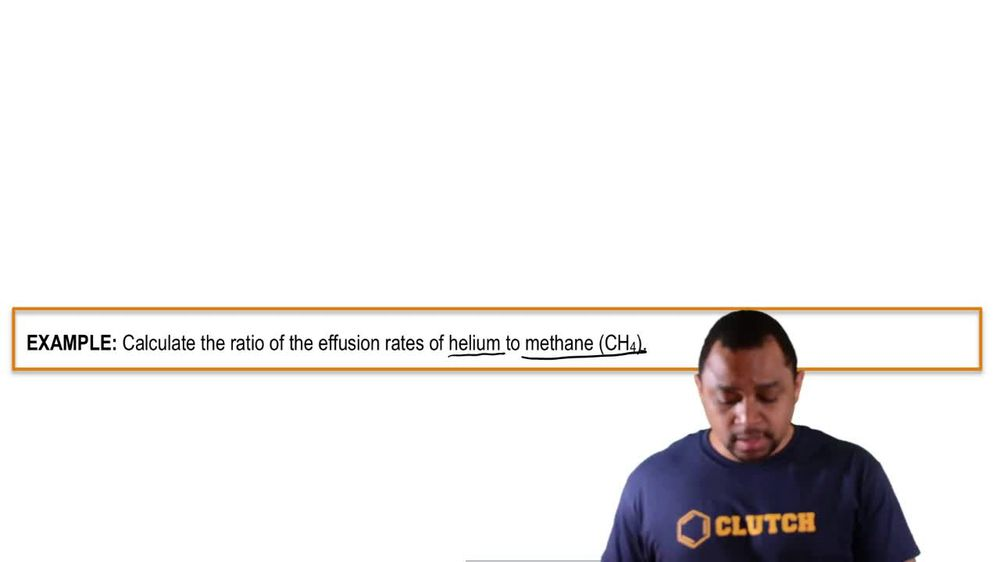Start typing, then use the up and down arrows to select an option from the list.
1. 7. Gases2. Effusion# Effusion Example 2

by Jules Bruno
116 views
1
1
here. The example, Question says, calculate the ratio of the infusion rates of helium to methane. All right, so the first gas that's name represents our gas A and the second one will represent our gas. Be so here. We're going to say, rate off helium divided by the rate off methane. We were not given times for them, so we can't use time in terms of this question. But we know the identity of the gas is therefore we know they're Moeller masses. So this equals. Remember Right and Mueller master inversely proportional. So if the rate of helium is on the top than the molar, mass of helium has to be on the bottom because the rate of methane is on the bottom, the molar mass of methane has to be on top. Here we have one carbon and four hydrogen when you add all of their masses together from the periodic table, will get its mass as 16.42 g per mole for methane. When you look up the atomic massive healing on the periodic table, it's 4.3 g per mole for helium, So divide 16.42 by 4. and take the square root, and that gives us approximately 2. So this would be the ratio in terms of the rate of effusions for helium to methane. Now, what is this number telling us Well, what this number is telling us. It's telling us that helium moves twice as fast as methane, and this makes sense because helium weighs less. Remember, we said that the less you weigh, the faster you're going to be able to move as a gas because methane ways mawr, it makes sense. It's not gonna be able to move quite as fast as helium. So again, we're figuring out the rate of gas and a gas be whatever's discussed versus gas a sec. One is gas be the answer is telling us how much faster the top gas in this case helium is to methane